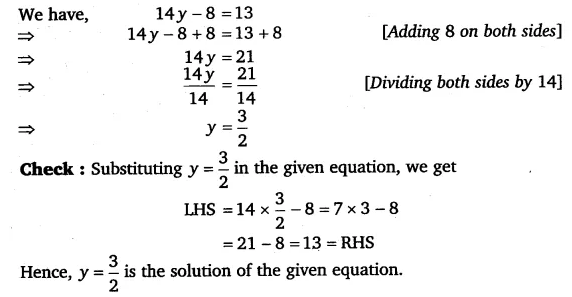# Solve the following equations: 14y – 8 = 13

Solve the following equations:
14y – 8 = 13